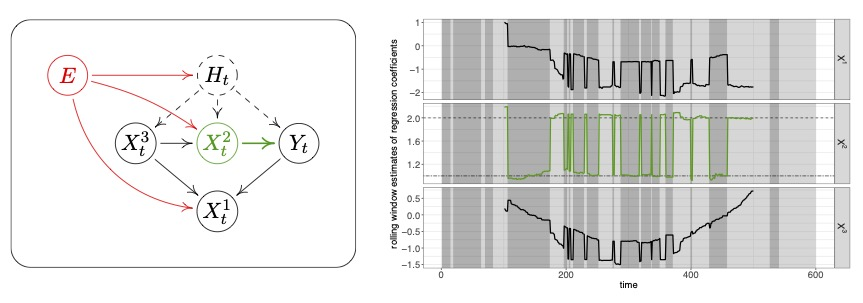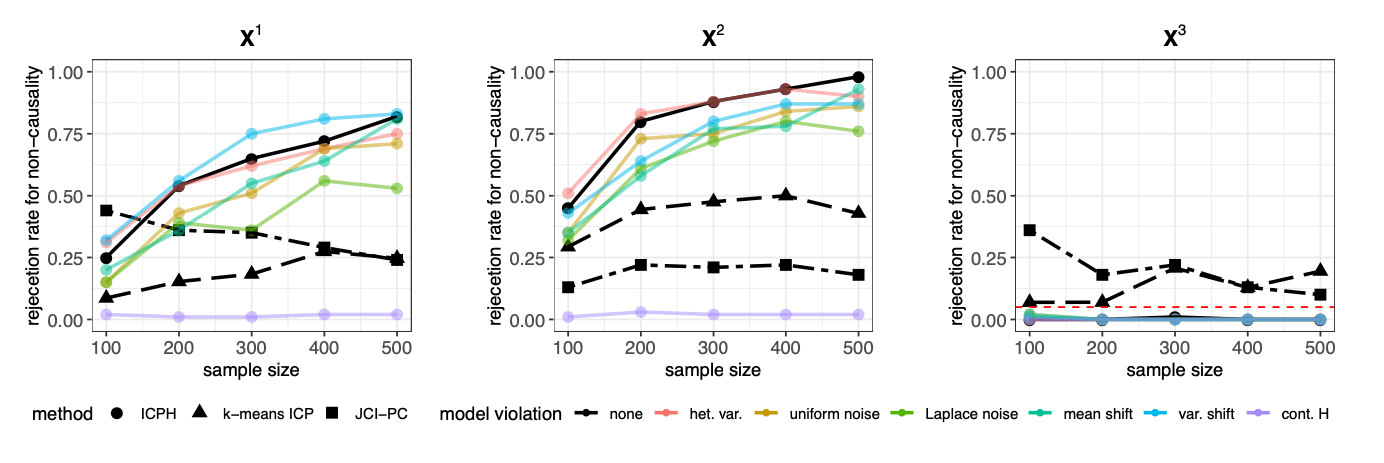Want to share your content on R-bloggers? click here if you have a blog, or here if you don't.

We address the problem of causal structure learning in the presence of hidden variables. Given a target variable and a vector of covariates, we are trying to infer the set of observable causal parents of the target variable. There are many good reasons for being interested in causal predictors.

Given a target variable $Y$, and a vector $X = (X^1, \dots, X^d)$ of $d$ covariates, we are trying to infer the set $S^* \subseteq \{1, \dots, d\}$ of observable causal parents of $Y$. There are many good reasons for being interested in causal predictors. One of them is the following well-known stability property: a regression model which regresses $Y$ on all of its causal parents remains valid under arbitrary interventions on any variable other than $Y$ itself. In cases where data are heterogeneous (e.g., in a time series setting), causal regression models can thus sometimes be used to obtain stable predictions across all different patterns of heterogeneity (e.g., across time). This stability property has been well-studied and is known under different names, e.g., invariance, autonomy or modularity , , .

In a setting without hidden variables, the invariance property has been exploited to recover (parts of) $S^*$: given data from a heterogeneous time series, one runs through all possible subsets $S \subseteq \{1, \dots, d\}$ and checks whether the conditional of $Y_t$ given $X_t^S := (X_t^j)_{j \in S}$ remains invariant across time. If more than one set satisfies invariance, one outputs the intersection of all invariant sets; this algorithm is known as the ICP (‘Invariant Causal Prediction’) algorithm . Here, we extend ICP to allow for unobserved direct causes of $Y$.

### Invariance and hidden variables

Let $(\mathbf{X}, \mathbf{Y}, \mathbf{H}) = (X_t,Y_t,H_t)_{t=1}^n \in \mathbb{R}^{n \times (d+1+1)}$ come from a structural causal model (SCM). Assume there exists a set $S^* \subseteq \{1, \dots, d\}$, a function $f$, and i.i.d. variables $(\varepsilon_t)_{t=1}^n \perp (\mathbf{X}, \mathbf{H})$, such that for all $t$, the assignment for $Y_t$ is given as

$$Y_t := f(X_t^{S^*}, H_t, \varepsilon_t).$$

All remaining structural assignments are allowed to vary across time. Our goal is to infer the unknown set $S^*$ based on a sample from $(\mathbf{X}, \mathbf{Y})$. Due to the hidden variables $H_t$ (whose distribution may vary over time), the conditional $P_{Y_t \vert X_t^{S^*}}$ is not ensured to be time-invarariant. However, the set $S^*$ satisifes a different form of invariance: using the law of total probability, it follows that for all $x,t$,

$$P_{Y_t \vert (X_t^{S^*} = x)} = \int \underbrace{P_{Y_t \vert (X_t^{S^*} = x, H_t = h)}}_{\text{invariant in } t} P_{H_t \vert (X_t^{S^*} = x)} (dh).$$

That is, $P_{Y_t \vert (X_t^{S^*} = x)}$ is equal to a mixture of several distributions $P_{Y_t \vert (X_t^{S^*} = x, H_t = h)}$, each of which is invariant in $t$, but with an associated mixture distribution $P_{H_t \vert (X_t^{S^*} = x)}$ which may change across time. We refer to this property as $h$-invariance (for ‘hidden-invariance’). Ideally, we would like to directly exploit this property for causal discovery, by running through all subsets $S \subseteq \{1, \dots, d\}$ and testing whether they satisfy $h$-invariance. To obtain statistical tests with any reasonable amount of power, we further impose the following simplifying assumptions. We assume that

• the hidden variables $H_t$ take only few different values $\{1, \dots, \ell\}$, and
• for every $h$, $f(\cdot, h, \cdot)$ is linear, and
• the noise variables $\varepsilon_1, \dots, \varepsilon_n$ are Gaussian.

Under these assumptions, the above integral reduces to a discrete sum of time-invariant linear Gaussian regressions. In other words, for $S = S^*$, the following null hypothesis holds true.

$H_{0,S}: \left\{ \begin{array}{l} \text{there exists } \theta = (\beta_j, \sigma_j^2)_{j=1}^\ell \text{ such that for all } t, P_{Y_t \vert X_t^S} \text{ is a mixture} \\ \text{of } \ell \text{ linear Gaussian regressions with regression parameters } \theta. \end{array} \right.$

Such mixtures of regressions are also known as “switching regression” models , . The above hypothesis specifies an invariance only in the mixing components of the model, while the mixing proportions are allowed to vary over time (this allows for the hidden variables to be heterogeneous). Given a family of statistical tests for $(H_{0,S})_{S \subseteq \{1, \dots, d\}}$, we can construct a causal discovery algorithm analogously to ordinary ICP.

### Algorithm: ICPH

Our causal discovery algorithm ICPH (‘Invariant Causal Prediction in the presence of Hidden variables’) operates in the following way.

• Use the sequential ordering of data to construct (disjoint) environments $e_1 \cup \cdots \cup e_k = \{1, \dots, n \}$.
• For each $S \subseteq \{1, \dots, d \}$, test $H_{0,S}$ using the environments.
• Output the intersection $\hat S := \bigcap_{S: H_{0,S} \text{ not rej.}} S$.

The main work is contained in the construction of valid tests of the hypotheses $H_{0,S}$. Given a candidate set $S \subseteq \{1, \dots, d\}$, we first fit a switching regression model to each environment $e_1, \dots, e_k$ separately. For each environment, we then compute a joint confidence region for the vector $\theta$ of regression parameters, and check whether all these regions have a non-empty intersection. We prove that this test obtains asymptotically valid level under mild assumptions. As a corollary, we directly obtain the asymptotic false discovery control of ICPH:

$$\text{for any test level } \alpha \in (0,1), \text{ we have } \lim \inf_{n \to \infty} P(\hat S_n \subseteq S^*) \geq 1-\alpha.$$

### Example

We now illustrate our method in the simple case where $d=3$ and $\ell=2$. Here, the target variable $Y_t$ is directly affected by $X_t^2$ and by the binary latent variable $H_t$. The structural assignment for $Y_t$ remains the same for all $t$, whereas some of the remaining assignments change continuously throughout time.The left figure illustrates the causal structure among $(X_t, Y_t, H_t)$ for each time point $t$. The node $E$ denotes the “environment variable” and indicates which of the structural assignments change over time. The assignment for $Y_t$ is the same for all observations, and the set $S^* = \{2\}$ is therefore $h$-invariant. This can be seen in the plot on the right, which shows rolling-window estimates of the regression coefficients for the regression of $Y_t$ onto each of the three predictors. Within both regimes $H_t = 1$ and $H_t = 2$ (corresponding to different background colors in the plot), the regression coefficient for $X_t^2$ (green) is time-homogeneous. When regressing $Y_t$ onto $X_t^1$ or $X_t^3$, the regression coefficients change in a more complicated fashion.

To infer $S^*$, our causal discovery algorithm now runs through all subsets $S \subseteq \{1,2,3\}$, and checks whether $H_{0,S}$ holds true. In this example, the results (assuming no test errors) are:so we correctly output $\hat S = \{2\} \cap \{2,3\} = \{2\}$. In general, we are not guaranteed to recover the full set $S^*$, but only a subset hereof.

### Empirical results

We test our algorithm on simulated data. Below, we show results of a simulation study where $X^1$ and $X^2$ are the true causal parents of $Y$, and $X^3$ is descendant of $Y$. For increasing sample size, we report, for each individual variable, the empirical frequency of inclusion in $\hat S$.Circles correspond to our method, while rectangles and triangles are two alternative methods that are included as baseline. Colored curves correspond to various model violations. As the sample size increases, ICPH tends to identify both causal parents $X^1$ and $X^2$, while the false discovery rate (the rate of inclusion of $X^3$) is controlled at level $\alpha = 0.05$. Our algorithm is robust against most considered model violations. If we allow the hidden variable to be continuous (purple curve), our method mostly returns the uninformative output $\hat S = \emptyset$.

### Reconstruction of the hidden variables

By assumption, the causal effect $X_t^{S^*} \to Y_t$ depends on the value of the hidden variable $H_t$. For example, $X_t^{S^*}$ may have a strong positive impact on $Y_t$ for $H_t = 1$, while $X_t^{S^*}$ and $Y_t$ are rendered entirely independent for $H_t=1$. In some applications, these differences in causal dependence are of particular interest in themselves, because they signify fundamentally different ‘states’ or ‘regimes’ of the underlying system. In such cases, we may thus want to reconstruct the hidden variables. If the true set of causal parents is known (e.g., because there is only one invariant set), we can estimate $\hat H_t = \operatorname{argmax}_{j \in \{1, \dots, \ell\}} \hat P(H_t = j \, \vert \, X_t^{S^*}, Y_t)$ directly from the fitted switching regression model $\hat P$ for $Y_t \, \vert \, X_t^{S^*}$. In the main paper, we apply this reconstruction approach to a real-world data set related to photosynthetic activity of terrestrial ecosystems, where the hidden variable corresponds to the vegetation type.

### This post is written by R. Christiansen and is based on

R. Christiansen and J. Peters. Switching regression models and causal inference in the presence of discrete latent variables. Journal of Machine Learning Research, 21(41):1−46, 2020.

Rune Christiansen is a postdoc at the Department of Mathematical Sciences, University of Copenhagen. He obtained his PhD degree from the same institution.

Jonas Peters is a professor at the Department of Mathematical Sciences, University of Copenhagen. Previously, he has been at the MPI for Intelligent Systems in Tuebingen and the Seminar for Statistics, ETH Zurich.

 J. Peters, P. Bühlmann, and N. Meinshausen. Causal inference by using invariant prediction: identification and confidence intervals. Journal of the Royal Statistical Society: Series B (Statistical Methodology), 78(5):947–1012, 2016.

 T. Haavelmo. The probability approach in econometrics. Econometrica, 12:S1–S115 (supplement), 1944.

 J. Aldrich. Autonomy. Oxford Economic Papers, 41:15–34, 1989.

 J. Pearl. Causality: Models, Reasoning, and Inference. Cambridge University Press, New York, USA, 2nd edition, 2009.

 R. De Veaux. Mixtures of linear regressions. Computational Statistics & Data Analysis, 8 (3):227–245, 02 1989.

 S. Goldfeld and R. Quandt. The estimation of structural shifts by switching regressions. Annals of Economic and Social Measurement, Volume 2, number 4, pages 475–485. NBER, 1973.